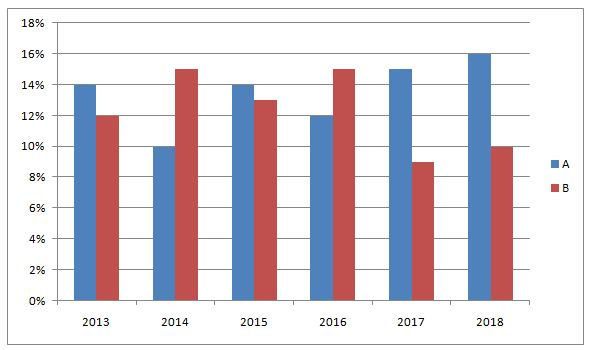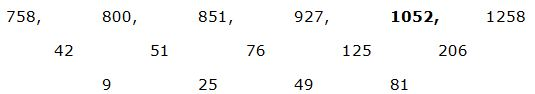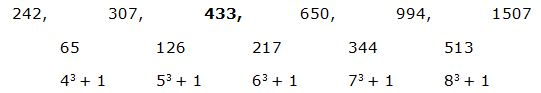# IBPS RRB PO Prelims Quantitative Aptitude Questions 2019 (Day-01)

Dear Aspirants, Our IBPS Guide team is providing new series of Quantitative Aptitude Questions for IBPS RRB PO Prelims 2019 so the aspirants can practice it on a daily basis. These questions are framed by our skilled experts after understanding your needs thoroughly. Aspirants can practice these new series questions daily to familiarize with the exact exam pattern and make your preparation effective.

Check here for IBPS RRB Prelims Mock Test 2019

[WpProQuiz 6459]

Directions (Q. 1 – 5): Find the wrong term in the following number series?

1) 758, 800, 851, 927, 1053, 1258

a) 1053

b) 1258

c) 927

d) 758

e) 851

2) 6, 7, 9, 18, 45, 135

a) 135

b) 45

c) 9

d) 7

e) 18

3)12, 14, 30, 94, 370, 1852

a) 370

b) 94

c) 30

d) 14

e) 1852

4) 242, 307, 432, 650, 994, 1507

a) 307

b) 1507

c) 432

d) 650

e) 994

5) 24, 12, 18, 47, 157.5, 708.75

a) 47

b) 18

c) 12

d) 157.5

e) 24

Directions (Q. 6 – 10): Study the following information carefully and answer the given questions.

The following bar graph shows the profit % earned by two different companies in 6 different years.6) If the expenditure of company A in the year 2013 is Rs. 138 lakhs, which is 25 % less than the income of company B in the year 2014, then find the sum of the income of company A in the year 2013 and theexpenditure of company B in the year 2014?

a) 317.32 lakhs

b) 378.46 lakhs

c) 405.74 lakhs

d) 456.18 lakhs

e) None of these

7) If the income of company A in the year 2015 and the income of company B in the year 2017 is same, find the ratio between the expenditure of company A in the year 2015 to that of the expenditure of company B in the year 2017?

a) 23 : 27

b) 57 : 62

c) 177 : 196

d) 109 : 114

e) None of these

8) If the income of company A in the year 2015 is 95 lakhs and the expenditure of company B in the year 2016 is 110 lakhs, the expenditure of company A in the year 2015 is approximately what percentage more/less than the income of company B in the year 2016?

a) 26 % more

b) 30 % less

c) 26 % less

d) 30 % more

e) 35 % less

9) If the expenditure of company A and B in the year 2018 is 125 lakhs and 140 lakhs respectively, then find the average income of company A and B in the year 2018?

a) 149.5 lakhs

b) 153.5 lakhs

c) 146 lakhs

d) 158 lakhs

e) None of these

10) If the expenditure of company A in the year 2017 is 120 lakhs and the income of company B in the year 2018 is 154 lakhs, then find the difference between the income of company A in the year 2017 to that of the expenditure of company B in the year 2018?

a) 5 lakhs

b) 6 lakhs

c) 2 lakhs

d) 4 lakhs

e) None of these

Directions (1-5):

The correct series is,The difference of difference is, 32, 52, 72, 92,…

The wrong term is, 1053

6*1 = 6

6*1.5 = 9

9*2 = 18

18*2.5 = 45

45*3 = 135

The wrong term is, 7

12*1 + 2 = 14

14*2 + 2 = 30

30*3 + 2 = 92

92*4 + 2 = 370

370*5 + 2 = 1852

The wrong term is, 94

The difference is, 43 + 1, 53 + 1, 63 + 1, 73 + 1, 83 + 1,…The wrong term is, 432

24*0.5 = 12

12*1.5 = 18

18*2.5 = 45

45*3.5 = 157.5

157.5*4.5 = 708.75

The wrong term is, 47

Directions (6-10):

The expenditure of company A in the year 2013 = Rs. 138 lakhs

The income of company A in the year 2013

= > 138*(114/100) = 157.32 lakhs

The income of company B in the year 2014 = 138*(100/75) = 184 lakhs

The expenditure of company B in the year 2014

= >184*(100/115) = 160 lakhs

Required sum = 157.32 + 160 = 317.32 lakhs

The income of company A in the year 2015 = The income of company B in the year 2017 = x

The expenditure of company A in the year 2015 = x*(100/114)

The expenditure of company B in the year 2017 = x*(100/109)

Required ratio = [x*(100/114)] : [x*(100/109)]

= >109 : 114

The income of company A in the year 2015 = 95 lakhs

The expenditure of company A in the year 2015

= >95* (100/114) = 83.33 lakhs

The expenditure of company B in the year 2016 = 110 lakhs

The income of company B in the year 2016

= > 110*(115/100) = 126.5 lakhs

Required % = [(126.5 – 88.33)/126.5]*100 = 30.17 % = 30 % less

The average income of company A and B in the year 2018

= > [125*(116/100) + 140*(110/100)]/2

= > [145 + 154]/2 = 149.5 lakhs

The expenditure of company A in the year 2017 = 120 lakhs

The Income of company A in the year 2017

= > 120*(115/100) = 138 lakhs

The income of company B in the year 2018 = 154 lakhs

The expenditure of company B in the year 2018

= > 154*(100/110) = 140 lakhs

Required difference = 140 – 138 = 2 lakhs